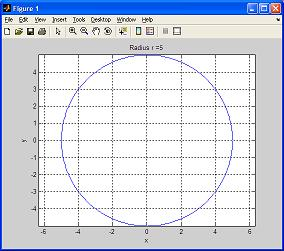# Tutorial Lesson: Matlab Programs (create and execute Function Files)

You'll learn to write and execute Matlab programs. Also, you'll learn the difference between a script file and a function file.

 A function file is also an M-file, just like a script file, but it has a function definition line on the top, that defines the input and output explicitly. You are about to create a MATLAB program! You'll write a function file to draw a circle of a specified radius, with the radius being the input of the function. You can either write the function file from scratch or modify the script file of this Tutorial Lesson.

Open the script file prettycircle.m (created and saved before).
On PCs, select 'Open' -> 'M-File' from the File menu. Make sure you're in the correct directory.

Navigate and select the file prettycircle.m from the 'Open' dialog box. Double click to open the file. The contents of the program should appear in an edit window.

Edit the file prettycircle.m from Tutorial 4 to look like the following:

function [x, y] = prettycirclefn(r)
% CIRCLE - A script file to draw a pretty circle
% Input: r = specified radius
% Output: [x, y] = the x and y coordinates

angle = linspace(0, 2*pi, 360);
x = r * cos(angle);
y = r * sin(angle);
plot(x,y)
axis('equal')
ylabel('y')
xlabel('x')
grid on

Now, write and save the file under the name prettycirclefn.m as follows:
On PCs, select 'Save As...' from the 'File' menu. A dialog box appears.

Type the name of the document as prettycirclefn. Make sure the file is saved in the folder you want (the current working folder/directory of MATLAB). Click on the 'Save' icon to save the file.

In the command window write the following:

>> prettycirclefn(5);

and you get the following figure (see the title):Then, try the following:

>>

and now you get a circle with radius = 3

Notice that a function file must begin with a function definition line. In this case is function [x, y] = prettycirclefn(r), where you define the input variables and the output ones.

The argument of the 'title' command in this function file is a combination of
a fixed part that never changes (the string 'Radius r= '), and a variable part that depends on the argumments passed on to the function, and that converts a number into a string (instruction 'num2str').

You can generate now a circle with an arbitrary radius, and that radius can be assigned to a variable that is used by your MATLAB program or function!

Great!

From 'Matlab Programs' to home

From 'Matlab Programs' to 'Matlab Tutorial'